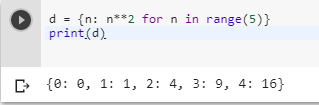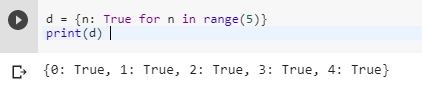3 views
in Python

Is it possible to create a dictionary comprehension in Python (for the keys)?

Without list comprehensions, you can use something like this:

l = []

for n in range(1, 11):

l.append(n)

We can shorten this to a list comprehension: l = [n for n in range(1, 11)].

However, say I want to set a dictionary's keys to the same value. I can do:

d = {}

for n in range(1, 11):

d[n] = True # same value for each

I've tried this:

d = {}

d[i for i in range(1, 11)] = True

However, I get a SyntaxError on the for.

In addition (I don't need this part, but just wondering), can you set a dictionary's keys to a bunch of different values, like this:

d = {}

for n in range(1, 11):

d[n] = n

Is this possible with a dictionary comprehension?

d = {}

d[i for i in range(1, 11)] = [x for x in range(1, 11)]

This also raises a SyntaxError on the for.

by (106k points)
edited by

If you want to create a dictionary comprehension in Python for that there are dictionary comprehensions in Python 2.7 and its upper versions. How they work is like a list comprehension, they create a new dictionary; they won’t add keys to an existing dictionary. And another thing you need to do is, you have to specify the keys and values.

d = {n: n**2 for n in range(5)}

print dAnother thing you are doing that you want to set them all to True so to do that you can use it as follows:

d = {n: True for n in range(5)}

print d